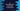# How to find the duplicate elements in an array in linear time# Introduction :

In this tutorial, we will learn how to find out the duplicate numbers in an array of positive integers in linear amount of time. This is one of the most commonly asked interview question. The array is given and its elements are in the range of 0 to array size - 1. i.e. for an array of size 4, its elements can be any one of 0, 1, 2 and 3. Elements can repeat and we need to find that.

In this tutorial, I will show you how to solve it in C, but you can use the same approach in any other programming language.

## Algorithm to use :

Two things we need to keep in mind:

• The array can contain only positive integers and
• It can contain integer from 0 to size - 1

Also, we are going to solve it in linear time, i.e. we will iterate through the array elements only once. Following are the steps used to find out the duplicates :

1. Initialize one empty array to hold the duplicate values. Scan through the array elements one by one starting from index 0.
2. For the current element, check the value in the array present at the absolute value of the current element index. For example, if the first element is 2 check for the element at index 2. If the first element is -3, check at index 3.
3. Check the value at that index. If it is positive, make it negative.
4. If the value is already negative, that means it was found before in the array. Add the index value to the array initialized for storing duplicate values.
5. Once the iteration will complete, the array will hold all duplicate elements.

## C program :

We can easily write the C program based on the above steps :

``````#include <stdio.h>
#include <stdlib.h>
#define SIZE 5

int main()
{
//1
int numArray[SIZE] = {2,3,3,5,4};
int duplicateArray[SIZE] = {-1,-1,-1,-1,-1};

//2
int i;
int k = 0;

//3
for(i = 0;i< SIZE;i++){
//4
if(numArray[abs(numArray[i])] < 0){
int val = abs(numArray[i]);
duplicateArray[k] = abs(numArray[i]);
k++;
}else{
//5
numArray[abs(numArray[i])] = -numArray[abs(numArray[i])];
}
}

//6
i = 0;
while(duplicateArray[i] != -1){
printf("%d ",duplicateArray[i]);
i++;
}
printf("\n");
return 0;
}``````

### Explanation :

The commented numbers in the above program denote the step numbers below :

1. numArray is the given array with numbers. We have initialized one more array duplicateArray to hold the duplicate numbers.
2. Initialize one variable i to use in the loop and k to define the current position in the duplicate element array.
3. Start one for loop to iterate through the elements in the array one by one.
4. Check the value at the position of the absolute value of the current element is positive or negative. If it is negative, append the index value to the duplicateArray.
5. If it is not negative, change it to negative.
6. After the for loop is completed, all duplicate values will be in the duplicateArray. Print them to the user.

### Output :

Based on the input array, it will print different outputs. For the above example, it will print 3 as the output. The time complexity for this solution is O(n).

### Conclusion :

In this tutorial, we have learned how to find the duplicate elements in an array in O(n) time. Try to run this program with different arrays and drop one comment if you have any queries.